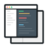# Taxi Trip Time Prediction using Regression, Numpy, Scipy in R

##### In this machine learning project , you will predict the total travel time of taxi trips from their initial partial trajectories.#### Videos

Each project comes with 2-5 hours of micro-videos explaining the solution.#### Code & Dataset## What will you learn

Understanding the problem statement
Importing the train and test dataset
Installing packages from R and GoogleMaps in R
Initializing important libraries and understanding its functions
Calculating distances in R using latitude and longitudinal points
Plotting scatter plots between different variables
Performing basic EDA and checking for null values
Imputing null values
Learning different techniques for imputing categorical and numeric variables
Changing character to factor vector in R
Removing unnecessary variables
Time stamping
Binning and visualizing time stamped data
Make a new dataset through data table
Selecting the model for training the pre-processed Dataset
Defining parameters for the model
Defining evaluation metrics for evaluating the model
Applying Random Forest model
Making the final predictions and saving it in CSV format

## Project Description

The goal of this machine learning project in R is to build a predictive model that can predict the total travelling time of 442 taxis running in the city of Porto based on their partial trajectories. This predictive framework will be used to enhance the efficiency of electronic taxi dispatching systems in Porto. You will use the taxi trajectory dataset from 01/07/2013 to 30/06/2014 containing the trajectories for all the 442 taxis running in the city of Porto.

## Similar Projects

#### Loan Eligibility Prediction using Gradient Boosting Classifier

This data science in python project predicts if a loan should be given to an applicant or not. We predict if the customer is eligible for loan based on several factors like credit score and past history.

#### Data Science Project in Python on BigMart Sales Prediction

The goal of this data science project is to build a predictive model and find out the sales of each product at a given Big Mart store.

#### Time Series Forecasting with LSTM Neural Network Python

Deep Learning Project- Learn to apply deep learning paradigm to forecast univariate time series data.

19-Dec-2015
03h 21m